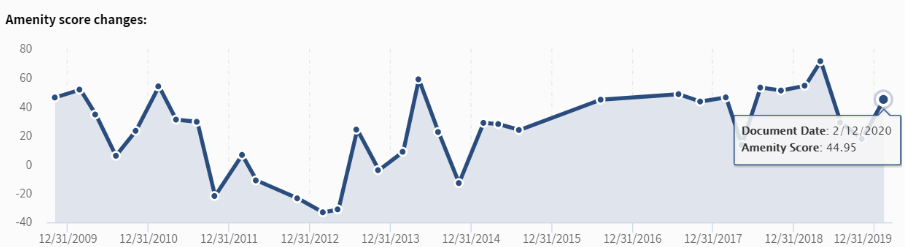# What is the Amenity Score?

The Amenity Score is a weighted average of all positive and negative events in a document represented by a trend line ending in a score of the most recent document. In presenting the change in the Amenity Score, we provide both the raw arithmetic difference between transcripts and the corresponding percentage change (Figure 1):Figure 1 Amenity Score

We calculate the percentage change with the following formula:

% change = (Current Amenity Score + 100) divided by (last month Amenity score + 100) – 1.

Note: The percentage change will never be less than -100% but can be well above 100%.